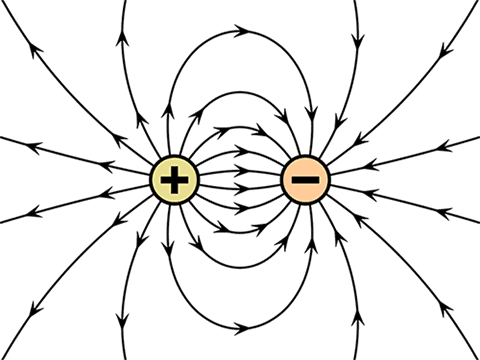# Electric charge

## What is Electric Charge in Physics?

In physics, Electric charge is the physical property of matter that causes it to experience a force when placed in an electromagnetic field. An electromagnetic field is a physical field produced by electrically charged objects. Electric charges are the lifeline of all the electronics we see around. The flow of current is due to the flow of electric charges.

The electric charge unit is Coulomb and it is denoted by ‘Q‘. The formula of the electric charge is given by the equation Q = I×t. Whereas ‘I’ is the current flowing through the conductor and ‘t’ is the amount of time the charge flows.

Electric charges can be measured by Galvanometer. There are three types of charges which are positive negative and neutral charges. An object said to be negatively charged if it has an excess number of electrons than protons.

On the other hand, if the object has an excess number of protons than electrons then the object is said to be positively charged. When the number of electrons and protons is equal then the object is said to be neutral.

## Electric charge carriers

Electric charge carriers are particles free to move to carry an electric charge. These electric charge carriers are present in electrical conductors so that the charges can pass through.

Electrons are charge carriers. Electron is a negatively charged particle. One or two of the valence electrons from each atom is above to move freely with the conductor. Proton is said to have a positive charge. However, it is not a charge carrier. Proton is an anti-electron. Proton lacks an electron and thus it acts as a positive charge but it is not.

And the particle with no net charge is the neutron. It is electrically neutral.

## Electric charges on an atom – Ions

Everything in this universe is made up of atom. Atom is the smallest unit of every material. An atom consists of electrons, protons, and a neutron. Protons and neutrons are packed together in the nucleus and electron revolves around the nucleus. Proton is a positively charged particle and the electron is a negatively charged particle.

In most of the atoms, the number of electrons and protons is equal, and thus net charge is zero. However, there are many atoms that either give away electrons or accept more electrons with relative ease. When this happens, we call the atom an ion.

If an electron goes away, then the atom is left with more protons than electrons, and the atom becomes a positive ion. Likewise, if an electron comes along and joins the atom, then there will be more electrons than there are protons, and the atom will become a negative ion.

## Coulomb’s law

The first law of Coulomb states that like charges repel and unlike charges attract each other. When opposite charges are kept near, then they will attract each other.

That means electron and proton attract each other while proton and proton repel each other.

The second law states that every charged particle experiences a force of attraction or repulsion from another charged particle. This force of attraction or repulsion is directly proportional to the magnitude of both the charges and inversely proportional to the square of the distance between them.

As the force is inversely proportional to the square of the distance. More the distance between the charged particles more will be the force and if the distance is more, the intensity of force would be less. Also, the force of attraction or repulsion depends on the medium in which the charges are placed.Mathematically, this law can be written as

##### And, F = k Q1 × Q2 / d2

Where,

k = Constant which changes its values depending on the medium where charges are placed.
Q1 and Q2 are the magnitude of both the charges.
d is the distance between the charges.
k can be replaced as

##### k = 1 / 4πɛ

Where ɛ is the permittivity of the medium.

The permittivity of every substance is different. The permittivity of the vacuum is taken as a base level and marked as 1. Then we would be able to get relative permittivity of other materials.

The base permittivity which is permittivity in a vacuum is denoted as ɛ˳ and relative permittivity is denoted as ɛr. Some permittivities of dielectric materials are

Air = 1.0006
Paper = 2.5 – 3.5
Wood = 3 – 8

The formula of actual permittivity is a product of base permittivity and relative permittivity.

##### ɛ = ɛ˳ × ɛr

Thus, we can rewrite the formula of k as

##### k = 1 / 4 π ɛ˳ ɛr

Thus, this is all about electric charges. Electric charges are the most fundamental topic in electricity physics. Electric charges are the only reason we can use electricity and of course we can’t even imagine this world without electricity. Our modern-day is dependent on electricity and from where it is generated by the flow of electric charges in a conductor.